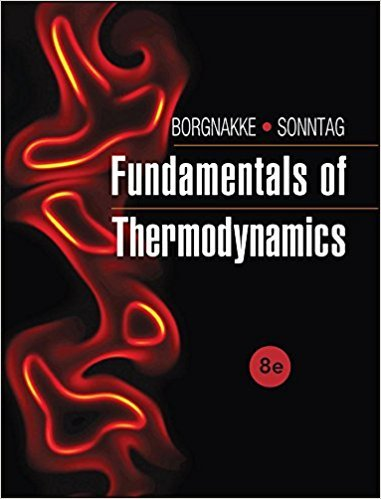×
Get Full Access to Fundamentals Of Thermodynamcs - 8 Edition - Chapter 5 - Problem 33hp
Get Full Access to Fundamentals Of Thermodynamcs - 8 Edition - Chapter 5 - Problem 33hp

×

# A large stationary diesel engine produces 5 MW with aISBN: 9781118131992 58

## Solution for problem 33HP Chapter 5

Fundamentals of Thermodynamcs | 8th Edition

• Textbook Solutions
• 2901 Step-by-step solutions solved by professors and subject experts
• Get 24/7 help from StudySoup virtual teaching assistantsFundamentals of Thermodynamcs | 8th Edition

4 5 1 237 Reviews
15
4
Problem 33HP

Problem 33HP

A large stationary diesel engine produces 5 MW with a thermal efficiency of 40%. The exhaust gas, which we assume is air, flows out at 800 K and the temperature of the intake air is 290 K. How large a mass flow rate is that, assuming this is the only way we reject heat? Can the exhaust flow energy be used?

Step-by-Step Solution:

Solution 33 HP

Step 1 of 6

We are required to calculate mass flow rate

Step 2 of 6

Step 3 of 6

##### ISBN: 9781118131992

Unlock Textbook Solution